About once a month I see a question on the SAS Support Communities that involves what I like to call "computations with combinations." A typical question asks how to find k values (from a set of p values) that maximize or minimize some function, such as "I have 5 variables, and for each observation I want to find the largest product among any 3 values."

These types of problems are specific examples of a single abstract problem, as follows:

1. From a set of p values, generate all subsets that contain k < p elements. Call the subsets Y1, Y2, ..., Yt, where t equals "p choose k". (In SAS, use the COMB function to compute the number of combinations: t = comb(p,k).)
2. For each subset, evaluate some function on the subset. Call the values z1, z2, ..., zt.
3. Return some statistic of the zi. Often the statistics is a maximum or minimum, but it could also be a mean, variance, or percentile.

This is an "exhaustive" method that explicitly generates all subsets, so clearly this technique is impractical for large values of p. The examples that I've seen on discussion forums often use p ≤ 10 and small values of k (often 2, 3, or 4). For parameters in this range, an exhaustive solution is feasible.

This general problem includes "leave-one-out" or jackknife estimates as a special case (k = p – 1), so clearly this formulation is both general and powerful. This formulation also includes the knapsack problem in discrete optimization. In the knapsack problem, you have p items and a knapsack that can hold k items. You want to choose the items so that the knapsack holds as much value as possible. The knapsack problem maximizes the sum of the values whereas the general problem in this article can handle nonlinear functions of the values.

### Example data

You can use the following DATA set to simulate integer data with a specified number of columns and rows. I use the relatively new "Integer" distribution to generate uniformly distributed integers in the range [-3, 9].

```%let p = 5; /* number of variables */ %let NObs = 6; /* number of observations */ data have(drop=i j); call streaminit(123); array x[&p]; do i = 1 to &NObs; do j = 1 to dim(x); x[j] = rand("Integer", -3, 9); /* SAS 9.4M4 */ end; output; end; ;   proc print data=have; run;```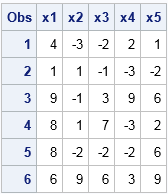### Computing with combinations in SAS/IML

For p = 5 and k = 3, the problem is: "For each observation of the 5 variables, find the largest product among any 3 values." In the SAS/IML language, you can solve problems like this by using the ALLCOMB function to generate all combinations of size k from the index set {1,2,...,p}. These values are indices that you can use to reference each combination of values. You can evaluate your function on each combination and then compute the max, min, mean, etc. For example, the following SAS/IML statements generate all combinations of 3 values from the set {1, 2, 3, 4, 5}:

```proc iml; p = 5; k = 3; c = allcomb(p, k); /* combinations of p items taken k at a time */ print c;```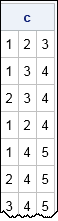A cool feature of the SAS/IML language is that you can use these values as column subscripts! In particular, the expression X[i, c] generates all 3-fold combinations of values in the i_th row. You can then use the SHAPE function to reshape the values into a matrix that has 3 columns, as follows:

```/* Example: find all combination of elements in the first row */ varNames = "x1":"x5"; use have; read all var varNames into X; close; Y = X[1, c]; /* all combinations of columns for 1st row */ M = shape(Y, nrow(c), k); /* reshape so each row has k elements */ prod = M[, #]; /* product of elements across columns */ print M prod;```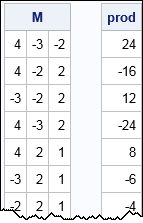Notice that each row of the matrix M contains k = 3 elements of Y. There are "5 choose 3" = 10 possible ways to choose 3 items from a set of 5, so the M matrix has 10 rows. Notice that you can use a subscript reduction operator (#) to compute the product of elements for each combination of elements. The maximum three-value product for the first row of data is 24.

The following loop performs this computation for each observation. The result is a vector that contains the maximum three-value product of each row. The original data and the results are then displayed side by side:

```/* for each row and for X1-X4, find maximum product of three elements */ result = j(nrow(X), 1); do i = 1 to nrow(X); Y = X[i, c]; /* get i_th row and all combinations of coloumns */ M = shape(Y, nrow(c), k); /* reshape so each row has k elements */ result[i] = max( M[,#] ); /* max of product of rows */ end; print X[colname=varNames] result[L="maxMul"];```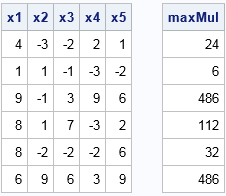Of course, if the computation for each observation is more complicated than in this example, you can define a function that computes the result and then call the module like this: result[i]= MyFunc(M);

### Generate all combinations in the DATA step

You can perform a similar computation in the DATA step, but it requires more loops. You can use the ALLCOMB function (or the LEXCOMBI function) to generate all k-fold combinations of the indices {1, 2, ..., p}. You should call the ALLCOMB function inside a loop from 1 to NCOMB(p, k). Inside the loop, you can evaluate the objective function on each combination of data values. Many DATA step functions such as MAX, MIN, SMALLEST, and LARGEST accept arrays of variables, so you probably want to store the variables and the indices in arrays. The following DATA step contains comments that describe each step of the program:

```%let p = 5; %let k = 3; %let NChooseK = %sysfunc(comb(&p,&k)); /* N choose k */ data Want(keep=x1-x&p maxProd); set have; array x[&p] x1-x&p; /* array of data */ array c[&k]; /* array of indices */ array r[&NChoosek]; /* array of results for each combination */ ncomb = comb(&p, &k); /* number of combinations */ do i=1 to &k; c[i]=0; end; /* zero the array of indices before first call to ALLCOMB */ do j = 1 to ncomb; call allcombi(&p, &k, of c[*]); /* generate j_th combination of indices */ /* evaluate function of the array {x[c], x[c], ..., x[c[k]]} */ r[j] = 1; /* initialize product to 1 */ do i=1 to &k; r[j] = r[j] * x[c[i]]; end; /* product of j_th combination */ end; maxProd = max(of r[*]); /* max of products */ output; run;   proc print data=Want; run;```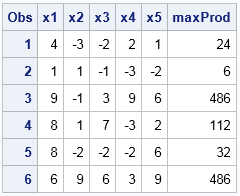The DATA step uses an array (R) of values to store the result of the function evaluated on each subset. For a MAX or MIN computation, this array is not necessary because you can keep track of the current MAX or MIN inside the loop over combinations. However, for more general problems (for example, find the median value), an array might be necessary.

In summary, this article shows how to solve a general class of problems. The general problem generates all subsets of size k from a set of size p. For each subset, you evaluate a function and produce a statistic. From among the "p choose k" statistics, you then choose the max, min, or some other measure. This article shows how to solve these problems efficiently in the SAS/IML language or in the SAS DATA step. Because this is a "brute force" technique, it is limited to small values of p. I suggest p ≤ 25.

ShareDistinguished Researcher in Computational Statistics

Rick Wicklin, PhD, is a distinguished researcher in computational statistics at SAS and is a principal developer of SAS/IML software. His areas of expertise include computational statistics, simulation, statistical graphics, and modern methods in statistical data analysis. Rick is author of the books Statistical Programming with SAS/IML Software and Simulating Data with SAS.

1.The code below illustrates two ways to solve this problem by using PROC OPTMODEL. Both ways use a binary variable for each element, along with a linear constraint that forces exactly k elements to be selected. The first approach uses the LSO solver (new in SAS Optimization 8.2) to maximize the product of selected elements. This way is straightforward but not guaranteed to find an optimal solution. The second approach uses a log transformation to linearize the product and then calls the MILP solver. As long as the maximum product is positive, this method is exact and scales much better than brute force.

```%let p = 5; /* number of variables */ %let NObs = 6; /* number of observations */ data have(drop=i j); call streaminit(123); array x[&p]; do i = 1 to &NObs; do j = 1 to dim(x); x[j] = rand("Integer", -3, 9); /* SAS 9.4M4 */ end; output; end; ;   proc print data=have; run;   %let k = 3;   /* LSO solver */ proc optmodel printlevel=0; /* declare parameters and read data */ num p = &p; num k = &k; set OBS; set ELEMENTS = 1..p; num x {OBS, ELEMENTS}; read data have into OBS=[_N_] {j in ELEMENTS} < x[_N_,j]=col('x'||j) >; num i_this;   /* Select[j] = 1 if element j is selected; 0 otherwise */ var Select {ELEMENTS} binary;   /* maximize product of selected elements */ /* max Product = prod {j in ELEMENTS} (if Select[j] = 1 then x[i_this,j] else 1);*/ max Product = prod {j in ELEMENTS} (x[i_this,j] * Select[j] + (1 - Select[j]));   /* select exactly k elements */ con Cardinality: sum {j in ELEMENTS} Select[j] = k;   /* FOR loop is serial, but COFOR loop is parallel */ set SELECTED = {j in ELEMENTS: Select[j].sol > 0.5}; num maxMul {OBS}; cofor {i in OBS} do; put i=; i_this = i; solve with LSO; print {j in SELECTED} x[i,j]; maxMul[i] = prod {j in SELECTED} x[i,j]; end; print maxMul; quit;     /* MILP solver */ proc optmodel printlevel=0; /* declare parameters and read data */ num p = &p; num k = &k; set OBS; set ELEMENTS init 1..p; num x {OBS, ELEMENTS}; read data have into OBS=[_N_] {j in ELEMENTS} < x[_N_,j]=col('x'||j) >; num i_this;   /* Select[j] = 1 if element j is selected; 0 otherwise */ var Select {ELEMENTS} binary;   /* maximize product of selected elements */ /* max Product = prod {j in ELEMENTS} (x[i_this,j] * Select[j] + (1 - Select[j]));*/ /* linearize by taking log */ max LogProduct = sum {j in ELEMENTS} log(abs(x[i_this,j])) * Select[j];   /* select exactly k elements */ con Cardinality: sum {j in ELEMENTS} Select[j] = k;   /* to get a positive product, select an even number of negative elements */ var Y >= 0 integer; con PositiveProduct: sum {j in ELEMENTS: x[i_this,j] < 0} Select[j] = 2 * Y;   /* FOR loop is serial, but COFOR loop is parallel */ set SELECTED = {j in ELEMENTS: Select[j].sol > 0.5}; num maxMul {OBS}; cofor {i in OBS} do; put i=; i_this = i; ELEMENTS = {j in 1..p: x[i_this,j] ne 0}; /* log(0) is undefined */ solve; print {j in SELECTED} x[i,j]; maxMul[i] = prod {j in SELECTED} x[i,j]; end; print maxMul; quit;```
2.Rick,
Since @RobPratt has already posted OR solution, I want to post another Data Step solution(no need build-in function),most likely Computer Science Student does.

%let p = 5; /* number of variables */
%let NObs = 6; /* number of observations */
data have(drop=i j);
call streaminit(123);
array x[&p];
do i = 1 to &NObs;
do j = 1 to dim(x);
x[j] = rand("Integer", -3, 9); /* SAS 9.4M4 */
end;
output;
end;
;
run;

data want;
set have;
array x{*} x1-x5;
max=.;
do i=1 to dim(x)-2;
do j=i+1 to dim(x)-1;
do k=j+1 to dim(x);
temp=x{i}*x{j}*x{k};
if temp>max then max=temp;
end;
end;
end;
drop temp i j k;
run;
proc print;run;

3.Sanja Jankovic on

How to write a sas code that finds and selects only the rows that sum to a given value in a dataset?

•You can ask SAS programming questions at the SAS Support Communities. Someone there might suggest that you use the SUM function in conjunction with the subsetting IF statement:

`if sum(of x1-x4) = 20; /* keep rows for which sum(x1,x2,x3,x4)=20 */`